# Solve the Differential problem? Find the general solution of the following system of differential Equations. Show...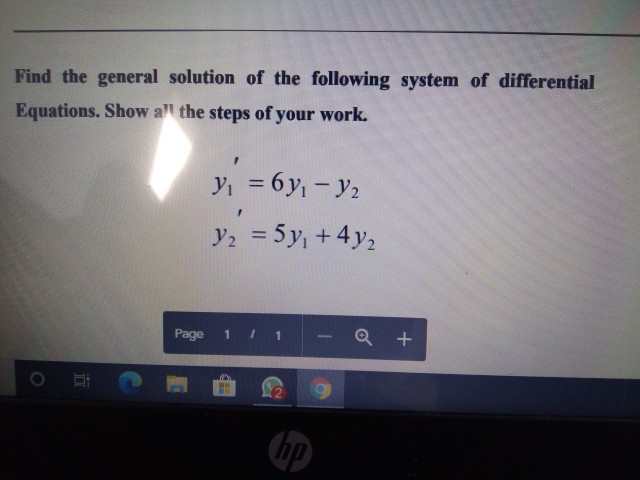Solve the Differential problem?

Find the general solution of the following system of differential Equations. Show a" the steps of your work. y = 6 yı - y2 V2 = 5y +442 Page 1 a + (hp)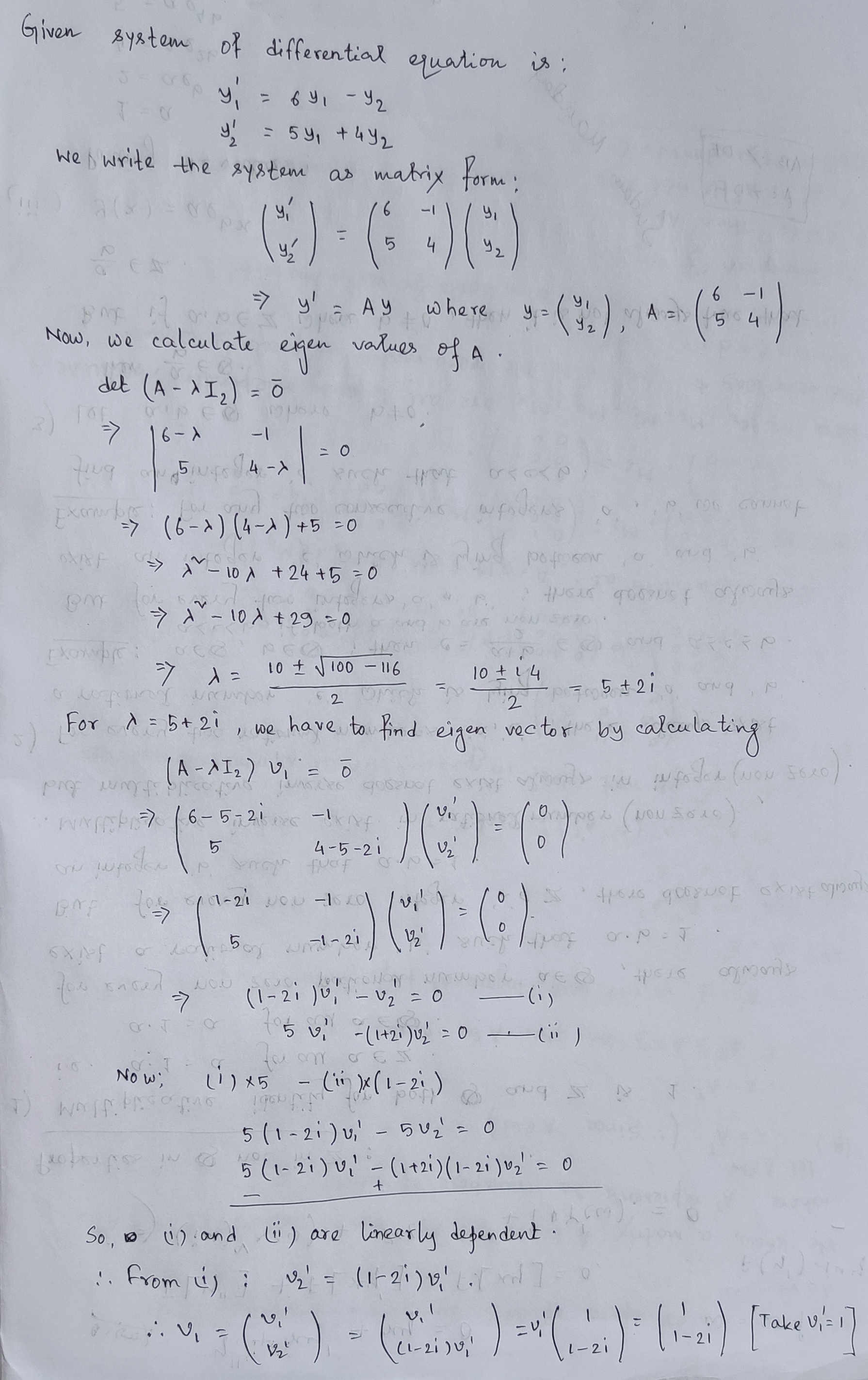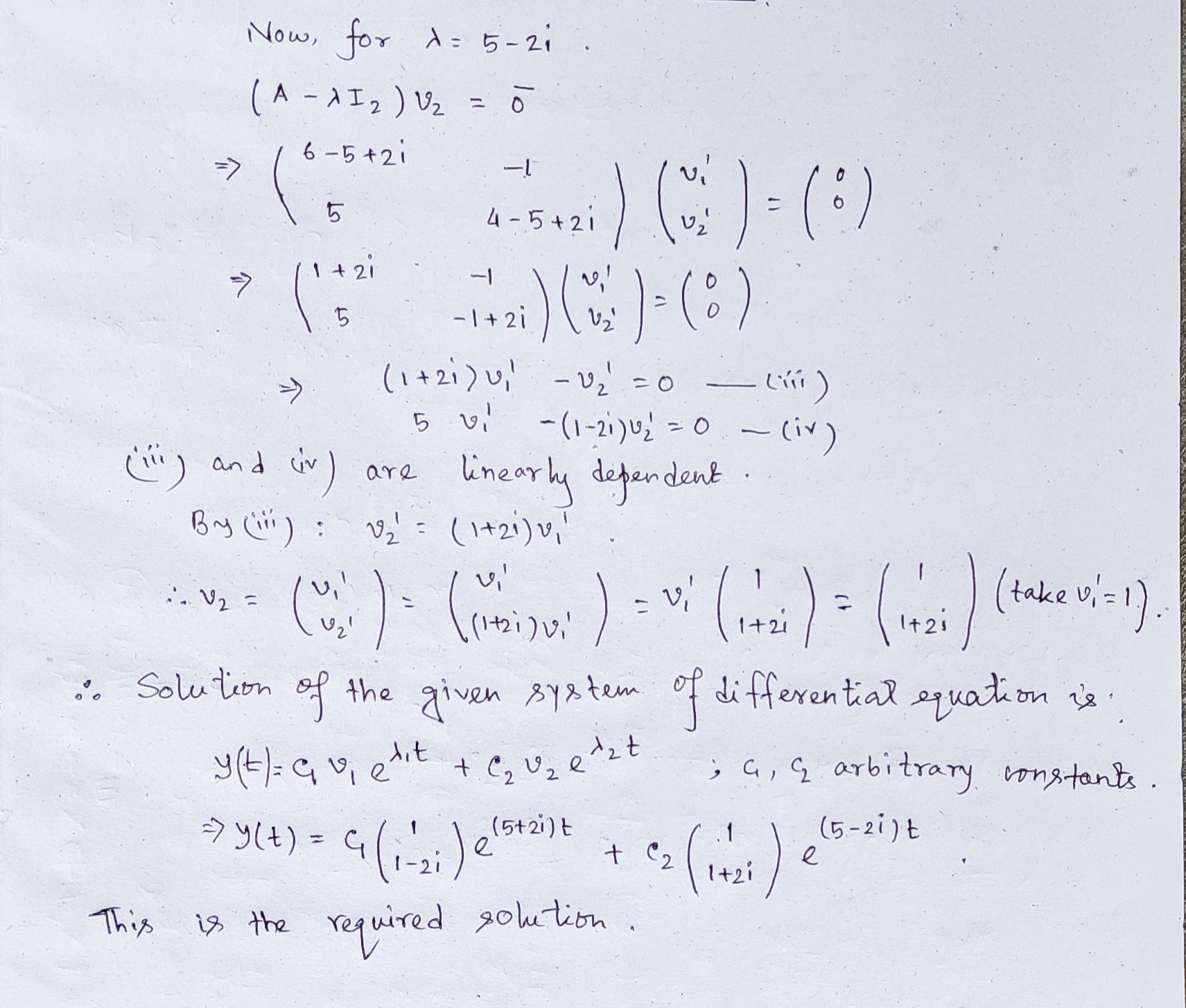##### Add Answer of: Solve the Differential problem? Find the general solution of the following system of differential Equations. Show...
Similar Homework Help Questions
• ### Find the general solution of the following system of differential Equations. Show all the steps of...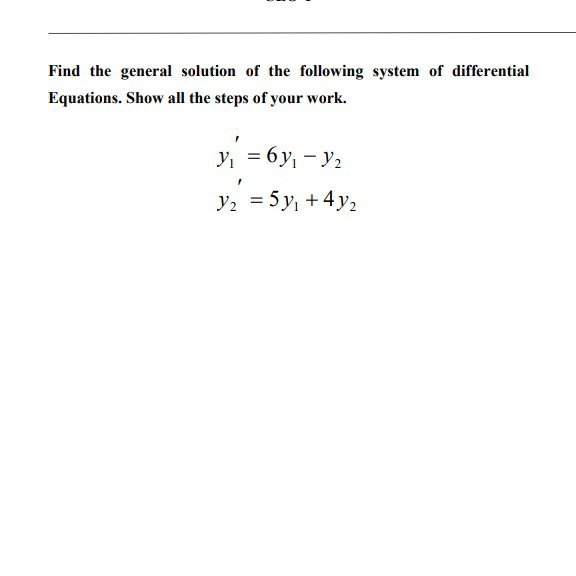Find the general solution of the following system of differential Equations. Show all the steps of your work. y = 6y; - y2 y2 = 5 y, +4y2

• ### 5. Find the general solution of the following differential equations: (a) 6"-5y y 0 (b) 4y"+12y9y...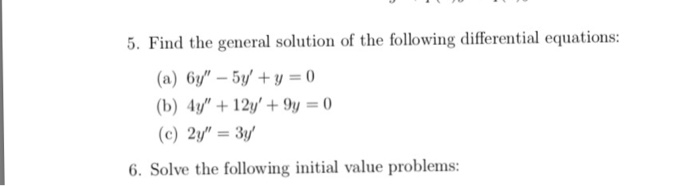5. Find the general solution of the following differential equations: (a) 6"-5y y 0 (b) 4y"+12y9y 0 (c)2" 3y 6. Solve the following initial value problems:

• ### Solve the system of differential equationsSolve the system of differential equations and find a particular solution if x(0)=1 and y(0)=-1.Use matrix methodPlease show your work

• ### non-homo 2nd order linear equations 1. Find the general solution for each of the following differential...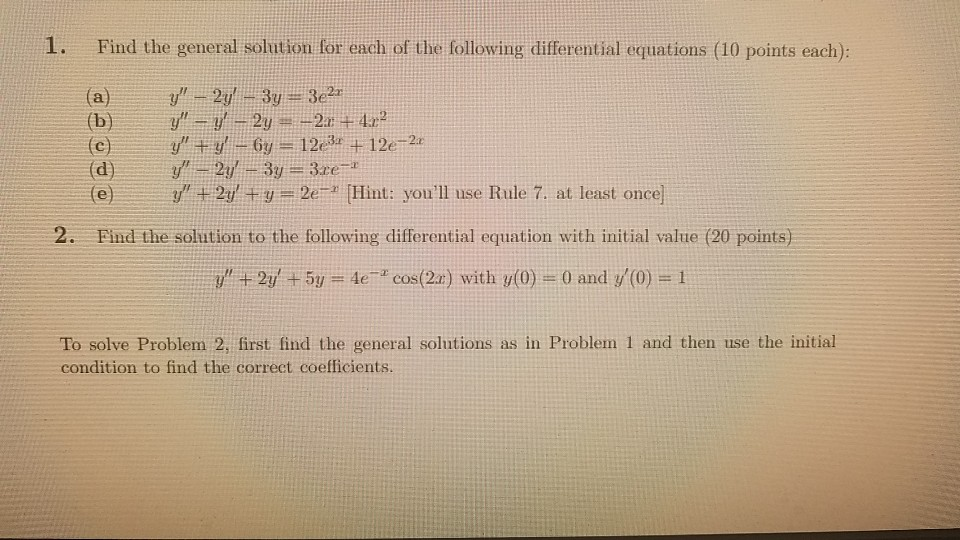non-homo 2nd order linear equations 1. Find the general solution for each of the following differential equations (10 points each): (a) (b) (e) y" – 2y! - 3y = 3e2x y" — y' – 2y = -2.3 + 4.2? y" + y’ – 67 = 1234 + 12e-2x y" – 2y' – 3y = 3.ce-1 y" + 2y' + y = 2e- (Hint: you'll use Rule 7. at least once) (e 2. Find the solution to the following differential equation...

• ### (1 point) Consider the initial value problem -2 j' = [ y, y(0) +3] 0 -2...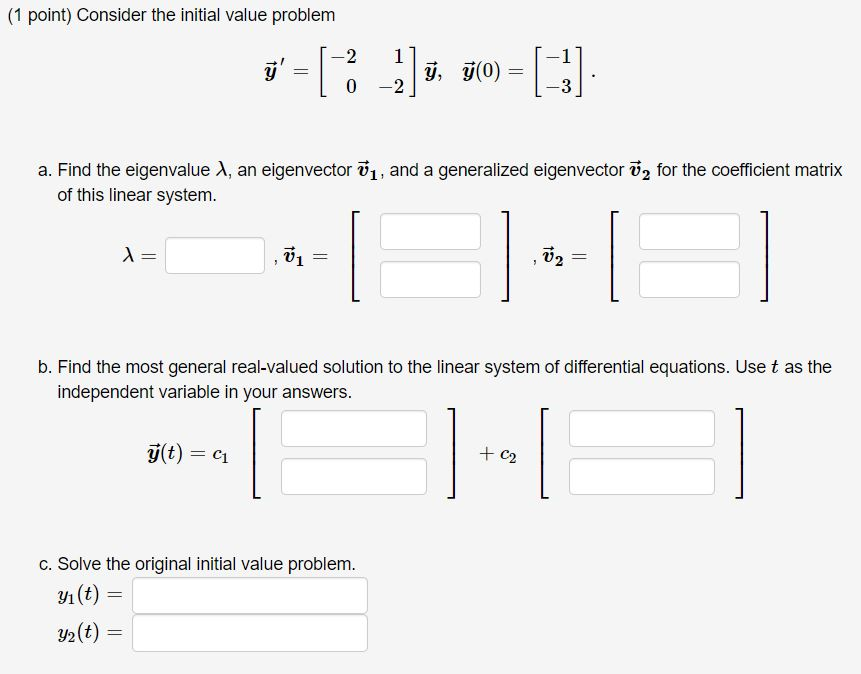(1 point) Consider the initial value problem -2 j' = [ y, y(0) +3] 0 -2 a. Find the eigenvalue 1, an eigenvector 1, and a generalized eigenvector ū2 for the coefficient matrix of this linear system. = --1 V2 = b. Find the most general real-valued solution to the linear system of differential equations. Use t as the independent variable in your answers. g(t) = C1 + C2 c. Solve the original initial value problem. yı(t) = y2(t) ==

• ### Find the general solution of the following differential equation: (1) ?′′ + 5?′ + 6? =...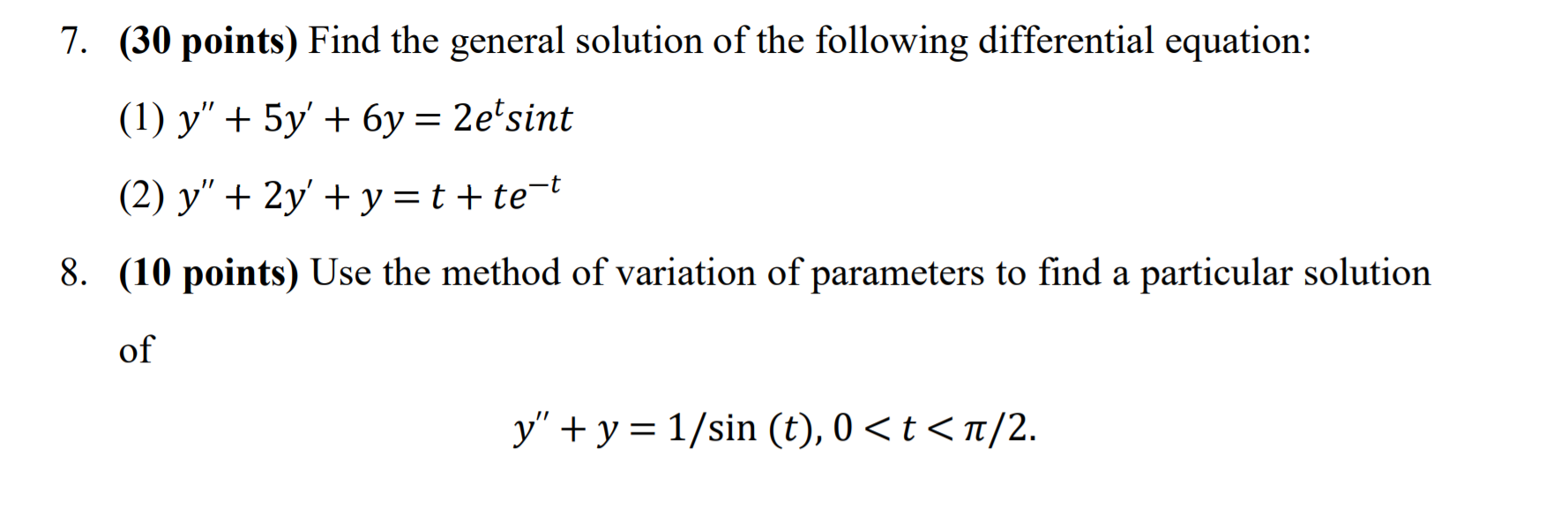Find the general solution of the following differential equation: (1) ?′′ + 5?′ + 6? = 2????*?^? (2) ?′′ + 2?′ + ? = ? + ?e^(-t). (please solve Question No.7 only) 7. (30 points) Find the general solution of the following differential equation: (1) y" + 5y' + 6y = 2etsint (2) y" + 2y + y=t+te-t 8. (10 points) Use the method of variation of parameters to find a particular solution of y" + y = 1/sin (t),...

• ### Step by step please. Solve the system of first-order linear differential equations. (Use C1 and C2...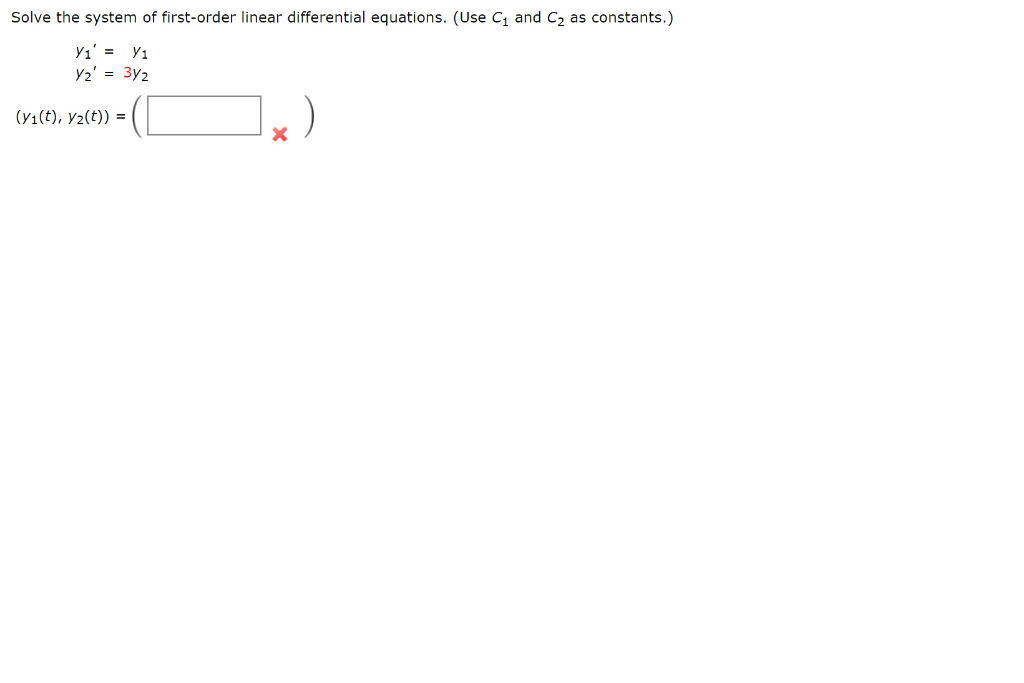Step by step please. Solve the system of first-order linear differential equations. (Use C1 and C2 as constants.) Yı' = y1 Y2' = 3y2 (y1(t), yz(t)) = ) x Solve the system of first-order linear differential equations. (Use C1, C2, C3, and C4 as constants.) Yi' = 3y1 V2' = 4Y2 Y3' = -3y3 Y4' = -474 (71(t), yz(t), y(t), 74(t)) =

• ### 2. Differential equations and direction fields (a) Find the general solution to the differential equation y'...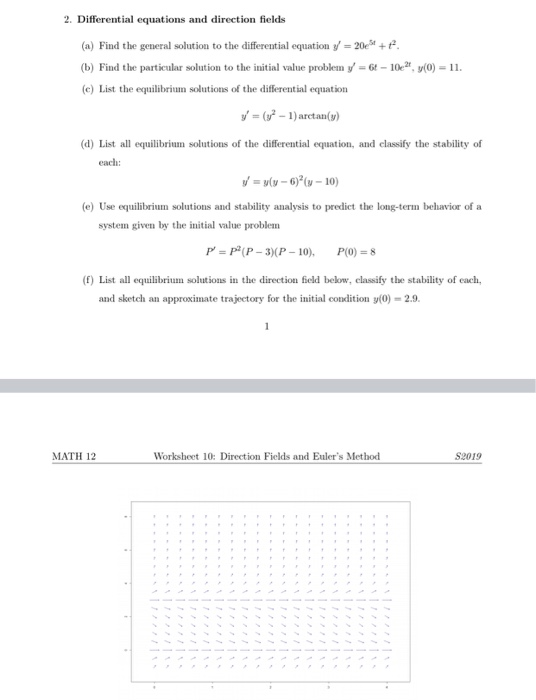2. Differential equations and direction fields (a) Find the general solution to the differential equation y' = 20e3+ + + (b) Find the particular solution to the initial value problem y' = 64 – 102, y(0) = 11. (e) List the equilibrium solutions of the differential equation V = (y2 - 1) arctan() (d) List all equilibrium solutions of the differential equation, and classify the stability of each: V = y(y - 6)(n-10) (e) Use equilibrium solutions and stability analysis...

• ### Problem 6. (1 point) Find the most general real-valued solution to the linear system of differential...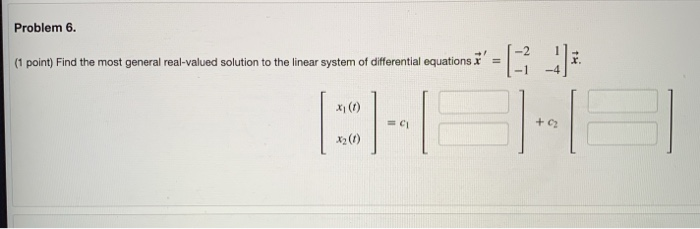Problem 6. (1 point) Find the most general real-valued solution to the linear system of differential equations & x (1) + C2

• ### (1 point) The system of first order differential equations y = -10yı -6y2 y = 12...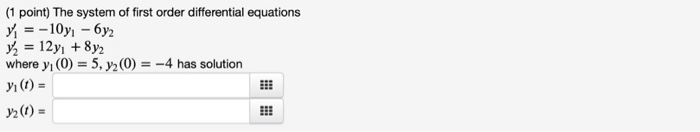(1 point) The system of first order differential equations y = -10yı -6y2 y = 12 yı + 8y2 where yı(O) = 5, y2(0) = -4 has solution yi (t) = y ) =

Free Homework App